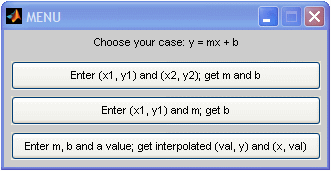# Line Segment - equation of a straight line in Matlab

A line segment is a straight line which links two points without extending beyond them. In this article we’re going to develop three functions to work out equations of straight lines in Matlab with different approaches.

 At the end, we’re going to wrap those three functions in a menu, so that the user can choose the appropriate one, according to known data. In two dimensions, the equation is often given by the slope-intercept form y = mx + b where:

m is the slope of the line (y2 – y1)/(x2 – x1)
b is the y-intercept of the line
x is the independent variable of the function y = f(x)Our first function assumes you enter points (x1, y1) and (x2, y2), and the function returns the slope m and the intersect b.

function [m, b] = str_lin1(x1, y1, x2, y2)
m = (y2-y1) / (x2-x1);
b = y1 - m*x1;

Now, the following video shows you how to use the concepts of point and slope to find linear equations. After the video, we show you how to implement a function to easily achieve the equivalent results.

Our second function in this task, assumes you enter point (x, y) and the slope. It returns the intercept.

function b = str_lin2(x1, y1, m)
b = y1 - m*x1;

The third function interpolates an entered value. You enter the slope, the intercept and the value. The function returns the interpolated values.

function [x, y] = str_lin3(m, b, val)
y = m*val + b;
x = (val - b) / m;

Now, we’re going to wrap those three functions using a menu and a switch structure:

% Functions on straight lines
clear all, clc, format compact, close all

'Enter (x1, y1) and (x2, y2); get m and b',...
'Enter (x1, y1) and m; get b',...
'Enter m, b and a value; get interpolated (val, y) and (x, val)');

switch option

case 1
x1 = input(
'Enter x1 = ');
y1 = input(
'Enter y1 = ');
x2 = input(
'Enter x2 = ');
y2 = input(
'Enter y2 = ');
[m, b] = str_lin1(x1, y1, x2, y2)

case 2
x1 = input(
'Enter x1 = ');
y1 = input(
'Enter y1 = ');
m = input(
'Enter m = ');
b = str_lin2(x1, y1, m)

case 3
m = input(
'Enter m = ');
b = input(
'Enter b = ');
v = input(
'Enter val = ');
[x, y] = str_lin3(m, b, v);
str = [
'(' num2str(v) ', ' num2str(y) ')'];
disp(str)
str = [
'(' num2str(x) ', ' num2str(v) ')'];
disp(str)

end

Let’s assume that the script above is called line_segment. We can run some examples from the command window, like this...

>>line_segment

### Example 1: enter two pointsIf we click on the first option, the flow is like this:

Enter x1 = 0
Enter y1 = -2
Enter x2 = 2/3
Enter y2 = 0

and the results are

m =  3
b = -2

### Example 2: enter one point and slope

Let's run again our code, but this time we select the second button. The scirpt works like this...

Enter x1 = 2/3
Enter y1 = 0
Enter m = 3

and the result is

b = -2

### Example 3: enter slope, intercept and one value to be interpolated

Finally, the third option can be used for a simple linear interpolation. Let's run it, and we get...

Enter m = -4
Enter b = 1
Enter val = 0

(0, 1)
(0.25, 0)

From 'Line Segment' to home

From 'Line Segment' to Flow Control